/

### The Beta distribution

The beta distribution describes a distribution in which outcomes are limited to a specific range, the probability density function within this range being characterised by two shape parameters.

 Distribution name Beta distribution Common notation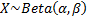Parameters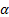= shape parameter (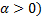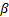= shape parameter (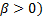Domain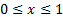Probability density function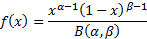Cumulative distribution function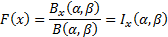Mean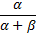Variance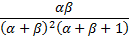Skewness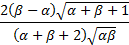(Excess) kurtosis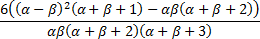Characteristic function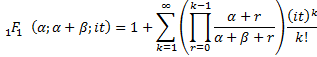Other comments The beta distribution is also known as a beta distribution of the first kind. Its mode is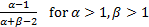. There is no simple closed form solution for its median.   The beta distribution parameters are sometimes taken to include boundary parameters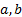(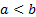) in which case its domain is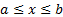, and its pdf and cdf are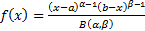and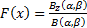where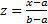, its mean is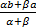, its mode is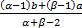for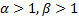and its variance is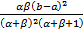If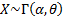and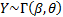then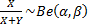. Ifthen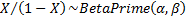and if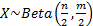then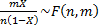(if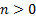and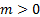). The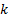’th order statistic of a sample of sizefrom the uniform distribution has a beta distribution,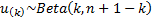.   If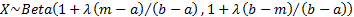then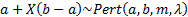, i.e. the Pert distribution is a special case of the beta distribution.   Its non-central moments are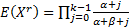.

Nematrian web functions

Functions relating to the above distribution may be accessed via the Nematrian web function library by using a DistributionName of “beta”. Functions relating to a generalised version of this distribution including additional location (i.e. shift) and scale parameters may be accessed by using a DistributionName of “beta4”, see also including additional shift and scale parameters. For details of other supported probability distributions see here.